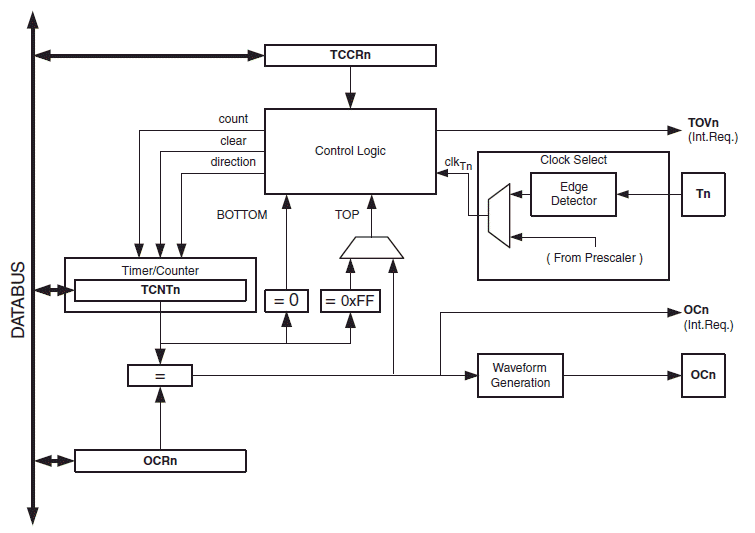# Normal Mode

Inputs:

Results:## About Arduino Timer 0 Calculator

The above online Normal Mode calculator helps you to calculate the timer/counter 0 count value(C) that is to be loaded into the TCNT0 register for given CPU frequency(oscillator frequency, FCPU) and required time delay(Td). It also calculates the CPU time period for given CPU frequency and the number of clocks or ticks that is required for specified time delay. This calculator can be used for making time delay routine for ATmega328P based Arduino board such as Arduino Uno and Arduino Nano.

### Equations Used:

$$C=256-\frac{F_{CPU}}{N F_{d}}$$
where, N is the pre-scalar value
$$F_d=\frac{1}{T_d}$$
where $$T_d$$ is the required time delay
The timer CPU period is, $$T_{CPU}=N\times(\frac{1}{F_{CPU}})$$
and the number of CPU clocks required for the specified time delay is, $$N_c=\frac{T_d}{T_{CPU}}$$

# CTC Mode

Inputs:

Results:## About Arduino Timer 0 CTC Mode Calculator

The above online CTC Mode calculator helps you to calculate the timer/counter 0 count value(C) that is to be loaded into the OCR0 register(Output Compare Register 0) for given CPU frequency(or oscillator frequency, Fosc) and desired output wave frequency(Fw).

### Equations Used:

$$C=\frac{F_{osc}}{2 N F_{w}}-1$$
where, Fosc is the frequency of the CPU, Fw is the output square wave frequency and N is the pre-scalar value.

# Fast PWM Mode

Inputs:

%

Results:

### Inverted Mode Fast PWM Mode:

Calculates OCR0, Wave Frequency & Wave Time Period
Inputs:

%

Results:## About Arduino Timer 0 Fast PWM Mode Calculator

The above online Fast PWM calculator calculates the wave frequency and count value to be loaded into OCR0 register for non-inverted and inverted mode.

### Equations Used:

$$F_{w(non-inv)}=\frac{F_{osc}}{256N},$$ $$OCR0_{non-inv} = \frac{256D}{100} - 1,$$ $$F_{w(inv)}=\frac{F_{osc}}{256N},$$ $$OCR0_{inv} = 255 - \frac{256D}{100}$$

# Phase Correct PWM Mode

Inputs:

%

Results:

### Inverted Mode Fast PWM Mode

Calculates OCR0, Wave Frequency & Wave Time Period
Inputs:

%

Results:## About Arduino Timer 0 Phase Correct PWM Mode Calculator

The above online Phase Correct PWM calculator calculates the wave frequency and count value to be loaded into OCR0 register for non-inverted and inverted mode.

### Equations Used:

$$F_{w(non-inv)}=\frac{Fosc}{510N},$$ $$OCR0_{non-inv}= \frac{255D}{100},$$ $$F_{w(non-inv)}=\frac{Fosc}{510N},$$ $$OCR0_{non-inv}= 255 - \frac{255D}{100}$$